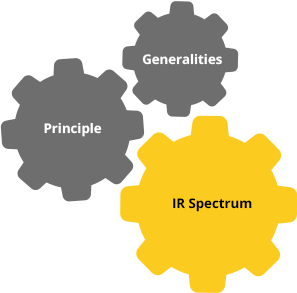## IR spectrum

An IR spectrum represents the intensity of IR radiation as a function of wave number.On the Y-axis: transmittance T or absorbance A

T = I/I0 in % with
I0 = light intensity measured without sample (background)
= contribution of the apparatus + environment
I = light intensity measured with a sample
= contribution of the apparatus + environment + sample
Thus I/I0 = sample contribution
A = log I0/I without unit

On the X-axis: the wavenumber, in cm-1

Click on each box to find out more

Most organic molecules are infrared active. However, two conditions are required to induce vibrations.A molecule absorbs IR light if it has a vibration during which its dipole moment varies with distance. It can be translated mathematically by the following relationship:The energy of the incident radiation must be equal to the energy difference between two vibrational levels of the molecule.h = 6,63. 10-34 J.S
c = 3 10.10 cm.s-1
ω = wavenumber in cm-1
Click on each box to find out more
Next page
Previous page